# IA Monitoring Earthquake Structures

Design and build a system for monitoring structural movements in smart buildings with Infineon 3D Magnetic 2Go.

IntermediateFull instructions provided2 hours6,477

## Things used in this project

### Hardware components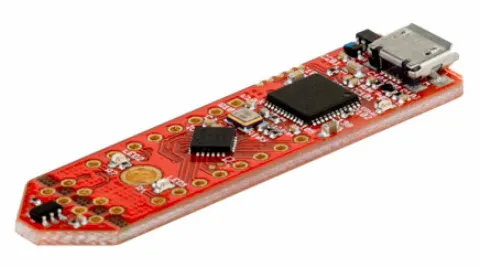Infineon 3D Magnetic Sensor 2Go
×1Arduino UNO
×1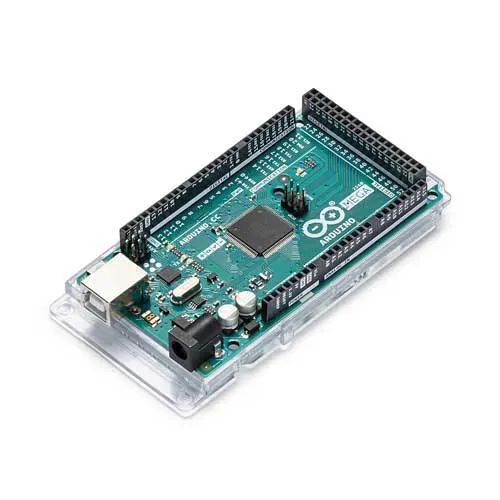Arduino Mega 2560
×1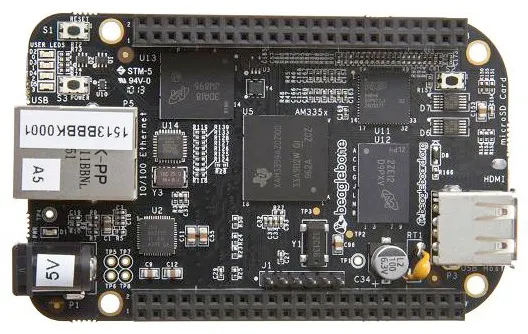BeagleBoard.org BeagleBone Black
×1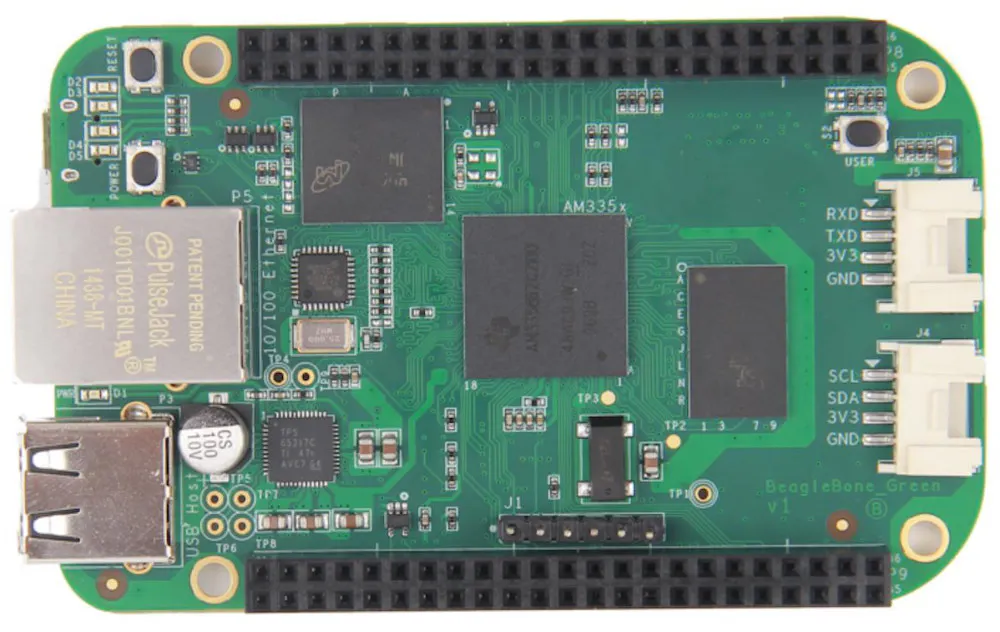BeagleBoard.org SeeedStudio BeagleBone Green
×1

### Software apps and online servicesArduino IDE
 Infineon 3D 2Go Shortcut
 jAmaSeisMicrosoft Windows 10
 Microsoft Office Excel 2016

### Hand tools and fabrication machines

 Welder
 Computer

## Schematics

### Arduino Diagram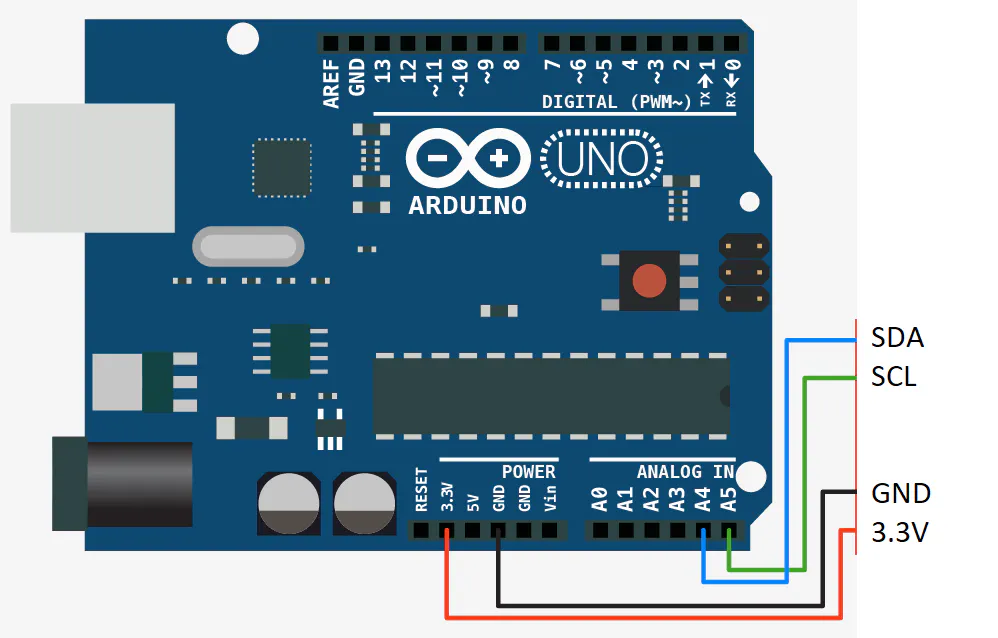### Beaglebone Diagram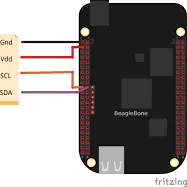## Code

### CODIGO_FINAL_INFINEON_SISMOMETRO.ino

C/C++
This is the final code to load on the Arduino board. It is still in test and improvements, if you identify errors or improvements please tell us.
```/* nitial sketch by Mark J. Hughes for AllAboutCircuits.com
* Modified and adapted for the sensor 3D Magnetic Sensor 2Go TLE493D A2B6
By: Gabriel A. Giraldo and Jeremy Patrick
*/

//--- Begin Includes ---//
#include <I2C.h>       // http://dsscircuits.com/articles/arduino-i2c-master-library

// Variable Declaration
const byte addr = 0x5E; // default address of magnetic sensor 0x5E, 0x3E or 0X1F
byte rbuffer;       // store data from sensor read registers
byte delaytime = 1;     // time to wait before next read
bool PRINT_RAW_VALUES = false;

//--- Begin Setup ---//
void setup() {
Serial.begin(115200);      // Begin serial connection for debug.
I2c.begin();              // Begin IC I2c communication
I2c.timeOut(100);
}
//--- End of Setup --//

//--- Begin Main Program Loop --//
void loop() {

delay(delaytime); // wait time between reads.
// Read sensor registers and store in rbuffer
for(int i=0; i < 7; i++){
}

// Goto decode functions below
int x = decodeX(rbuffer,rbuffer);
int y = decodeY(rbuffer,rbuffer);
int z = decodeZ(rbuffer,rbuffer);
int t = decodeT(rbuffer,rbuffer);

if(rbuffer & B00000011 != 0){ // If bits are not 0, TLV is still reading Bx, By, Bz, or T

}
else {
if(PRINT_RAW_VALUES){
Serial.print(x);
Serial.print("\t");
Serial.print(y);
Serial.print("\t");
Serial.print(z);
Serial.print("\t");
Serial.println(t);
}
else{
Serial.print(convertToMag(x));
Serial.print("\t");
Serial.print(convertToMag(y));
Serial.print("\t");
Serial.print(convertToMag(z));
Serial.print("\t");
Serial.println(convertToCelsius(t));
}
}

}
//-- End of Main Program Loop --//

//-- Begin Buffer Decode Routines --//
int decodeX(int a, int b){
/* Shift all bits of register 0 to the left 4 positions.  Bit 8 becomes bit 12.  Bits 0:3 shift in as zero.
* Determine which of bits 4:7 of register 4 are high, shift them to the right four places -- remask in case
* they shift in as something other than 0.  bitRead and bitWrite would be a bit more elegant in next version
* of code.
*/
int ans = ( a << 4 ) | (((b & B11110000) >> 4) & B00001111);

if( ans >= 2048){ ans = ans - 4096; } // Interpret bit 12 as +/-
return ans;
}

int decodeY(int a, int b){
/* Shift all bits of register 1 to the left 4 positions.  Bit 8 becomes bit 12.  Bits 0-3 shift in as zero.
* Determine which of the first four bits of register 4 are true.  Add to previous answer.
*/

int ans = (a << 4) | (b & B00001111);
if( ans >= 2048){ ans = ans - 4096;} // Interpret bit 12 as +/-
return ans;
}

int decodeZ(int a, int b){
/* Shift all bits of register 2 to the left 4 positions.  Bit 8 becomes bit 12.  Bits 0-3 are zero.
* Determine which of the first four bits of register 5 are true.  Add to previous answer.
*/
int ans = (a << 4) | (b & B00001111);
if( ans >= 2048){ ans = ans - 4096;}
return ans;
}

int decodeT(int a, int b){
/* Determine which of the last 4 bits of register 3 are true.  Shift all bits of register 3 to the left
* 4 positions.  Bit 8 becomes bit 12.  Bits 0-3 are zero.
* Determine which of the first four bits of register 6 are true.  Add to previous answer.
*/
int ans;
a &= B11110000;
ans = (a << 4) | b;
if( ans >= 2048){ ans -= 4096;}
return ans;
}

float convertToMag(int a){
return a * 0.098;
}

float convertToCelsius(int a){
return (a-320)* 1.1;
}
```

### FINAL CODE FOR BEAGLEBONE

Python
Code for BeagleBone translate from C ++ to Python by Jeremy Patrick.
```## Requires to fix the read function in python I2C MRAA, line 1128 :

#     """
#     read(I2c self, int length) -> int

#     Parameters
#     ----------
#     length: int

#     """

import time
import mraa
import struct

delaytime = 0.001

print("Initialising the sensor")

magSensor = mraa.I2c(2, True)

rbuffer = bytearray(*10)

magSensor.writeReg(0x00, 0x05)

print("Sensor set to low power mode")

def decodeX( a,  b):
ans = ( a << 4 ) | (((b & 0b11110000) >> 4) & 0b00001111)
if( ans >= 2048):
ans = ans - 4096
return ans

def decodeY( a,  b):
ans = (a << 4) | (b & 0b00001111)
if( ans > 2048):
ans = ans - 4096
return ans

def decodeZ( a,  b):
ans = (a << 4) | (b & 0b00001111)
if( ans >= 2048):
ans = ans - 4096
return ans

def decodeT( a,  b):
a &= 0b11110000
ans = (a << 4) | b
if( ans > 2048):
ans -= 4096
return ans

def convertToMag(a):
return a * 0.098

def convertToCelsius(a):
return (a-320)* 1.1

while (True):
time.sleep(delaytime)
x = decodeX(rbuffer,rbuffer)
y = decodeY(rbuffer,rbuffer)
z = decodeZ(rbuffer,rbuffer)
t = decodeT(rbuffer,rbuffer)

if((rbuffer & 0b00000011) != 0): # If bits are not 0, TLV is still reading Bx, By, Bz, or T
else:
#print("%d \t %d \t %d \t %d" % (x,y,z,t)) # Raw values
print("%f \t %f \t %f \t %f" % (convertToMag(x),convertToMag(y),convertToMag(z),convertToCelsius(t)))
```

## Credits

### Gabriel Alejandro Giraldo Santiago

11 projects • 76 followers
I am a young boy with ideal to achieve everything that I propose. Lover of Science, Technology and innovation.
Thanks to HACKING STEM MICROSOFT, Jeremy Patrick , and Mark J. Hughes.